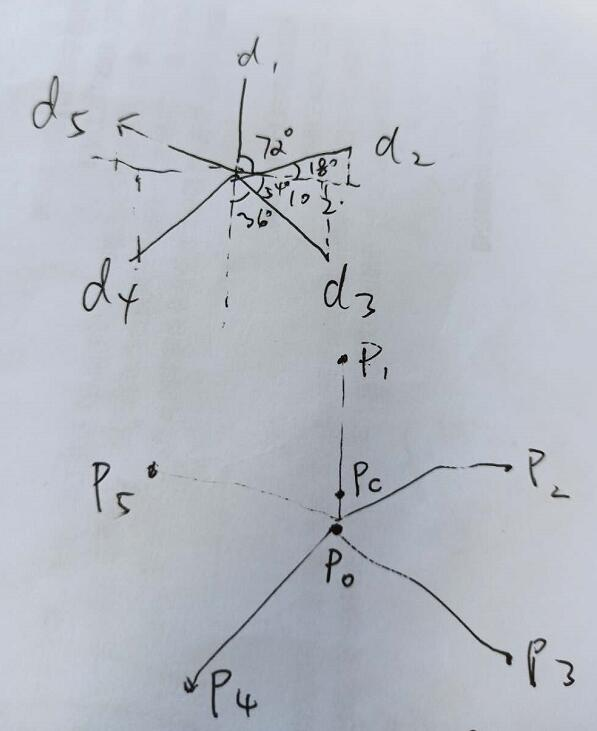# 小程序中使用 canvas 制作 5 维雷达图（结合小学六年级三角函数知识）

#### 实现思路#### 完整代码

``<canvas class="canvas" style="width: 210px; height: 200px;" canvas-id="canvas"></canvas>``

``````  onLoad () {
this.draw5DChart([0.8, 0.5, 0.7, 0.6, 1]) // 作图
}

draw5DChart (dArr) {
const get5dPoint = function (per, dim, w, h) {
const cos = Math.cos
const sin = Math.sin
const PI = Math.PI
const pc = [w / 2, h / 2]
const angleA = 90 - 360 / 5
const angleB = 90 - 360 / 5 / 2
const r = pc / cos(PI / 180 * angleA)
const p0 = [pc, r]
const p1 = [pc, 0]
const p2 = [pc * 2, p0 - r * sin(PI / 180 * angleA)]
const p3 = [pc + r * cos(PI / 180 * angleB), p0 + r * sin(PI / 180 * angleB)]
const p4 = [pc - r * cos(PI / 180 * angleB), p3]
const p5 = [0, p2]
switch (dim) {
case 1:
return [p1, p0 - p0 * per]
break
case 2:
return [p0 + (p2 - p0) * per, p0 - (p0 - p2) * per]
break
case 3:
return [p0 + (p3 - p0) * per, p0 + (p3 - p0) * per]
break
case 4:
return [p0 - (p0 - p4) * per, p0 + (p3 - p0) * per]
break
case 5:
return [p0 - p0 * per, p0 - (p0 - p5) * per]
break
default:
return null
}
}
const scale = 750 / wepy.getSystemInfoSync().windowWidth
const pArr = dArr.map((_, index) => {
return get5dPoint(_, index + 1, 210 / scale, 200 / scale)
})
pArr.push(pArr)
const context = wepy.createCanvasContext('canvas')
context.setStrokeStyle('#000000')
context.setFillStyle('rgba(255, 235, 59, 0.9)')
context.setLineWidth(1)
pArr.forEach((p, index) => {
if (index > 0) {
context.lineTo(p, p)
} else {
context.moveTo(p, p)
}
})
context.fill()
context.stroke()
context.draw()
}``````## 作者： 博主

Talk is cheap, show me the code!